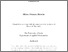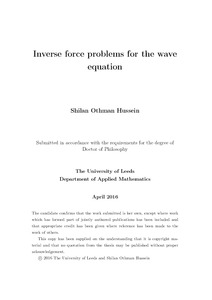# Inverse force problems for the wave equation

Hussein, Shilan Othman (2016) Inverse force problems for the wave equation. PhD thesis, University of Leeds.Preview
Text
Inverse force problems for the wave equation.pdf - Final eThesis - complete (pdf)

Download (6Mb) | Preview

## Abstract

Inverse problems have become more and more important in various fields of science and technology, and have certainly been one of the fastest growing areas in applied mathematics over the last three decades. However, as inverse problems typically lead to mathematical models which are ill-posed, their solutions are unstable under data perturbations and classical numerical techniques fail to provide accurate and stable solutions. The work in thesis focuses on inverse force problems for the wave equation which consists of determining an unknown space/time-dependent force function acting on a vibrating structure from Cauchy boundary, final time displacement or integral data. The novel contribution of this thesis involves the development of efficient numerical algorithms for these inverse but ill-posed problems. We have used the boundary element method (BEM) to discretise the wave equation with a constant wave speed, and the finite difference method (FDM) for non-constant wave speed and/or inhomogeneous wave propagating medium. Imposing the available boundary and additional conditions, upon discretisation the inverse and ill-posed problem is recast into one of solving an ill- conditioned system of equations. The accuracy and convergence of the numerical results are investigated for various test force functions. The stability of the numerical solutions is investigated by introducing random noise into the input data which yields unstable results if no regularisation is used. The Tikhonov regularization method is employed in order to reduce the influence of the measurement errors on the numerical results. The choice of the regularization parameter is based on trial and error or on the L-curve criterion. Iterative regularizing methods such as the Landweber and conjugate gradient methods are also employed in one chapter. The inverse numerical solutions are compared with their known analytical solutions, where available, and with the corresponding direct numerical solutions otherwise.

Item Type: Thesis (PhD) Inverse problem; Coefficient identification problem; Finite difference method; Wave equation; Nonlinear optimization; Finite-difference method; L-curve; Regularization The University of Leeds > Faculty of Maths and Physical Sciences (Leeds)The University of Leeds > Faculty of Maths and Physical Sciences (Leeds) > School of Mathematics (Leeds)The University of Leeds > Faculty of Maths and Physical Sciences (Leeds) > School of Mathematics (Leeds) > Applied Mathematics (Leeds) uk.bl.ethos.684540 Miss Shilan Hussein 27 Apr 2016 10:51 06 Oct 2016 14:42 http://etheses.whiterose.ac.uk/id/eprint/12653

You do not need to contact us to get a copy of this thesis. Please use the 'Download' link(s) above to get a copy.
You can contact us about this thesis. If you need to make a general enquiry, please see the Contact us page.